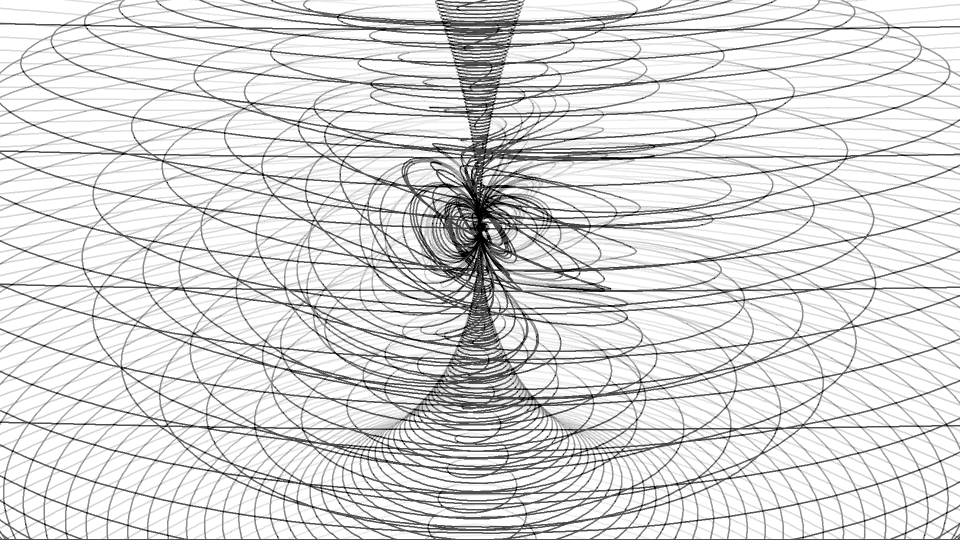index     sitemap     textsas animation     parametric form   this coarse graphic shows the 'unrolling line' of an increasing horn torusselected between ratios  v(φ) : v(ω) = 10 : 1  and  v(φ) : v(ω) = 1 : 1000 but not to scale and using rather rough differentials for the single steps, (otherwise the lines would lay very densely, according to the following:)    case r < 1: 1/r and φ increase with ω, starting with ω1 = 2π, according 1/r = ω / 2π (↝ r1 = 1) and φ = ω / r = ω2 / 2π (↝ φ1 = 2π), so we have x = (1 − cos(ω2 / 2π))·cosω·2π/ω y = (1 − cos(ω2 / 2π))· sinω·2π/ω z = sin(ω2 / 2π)· 2π/ωthe parametric forms show mirrored conditions,  compare  case r > 1: